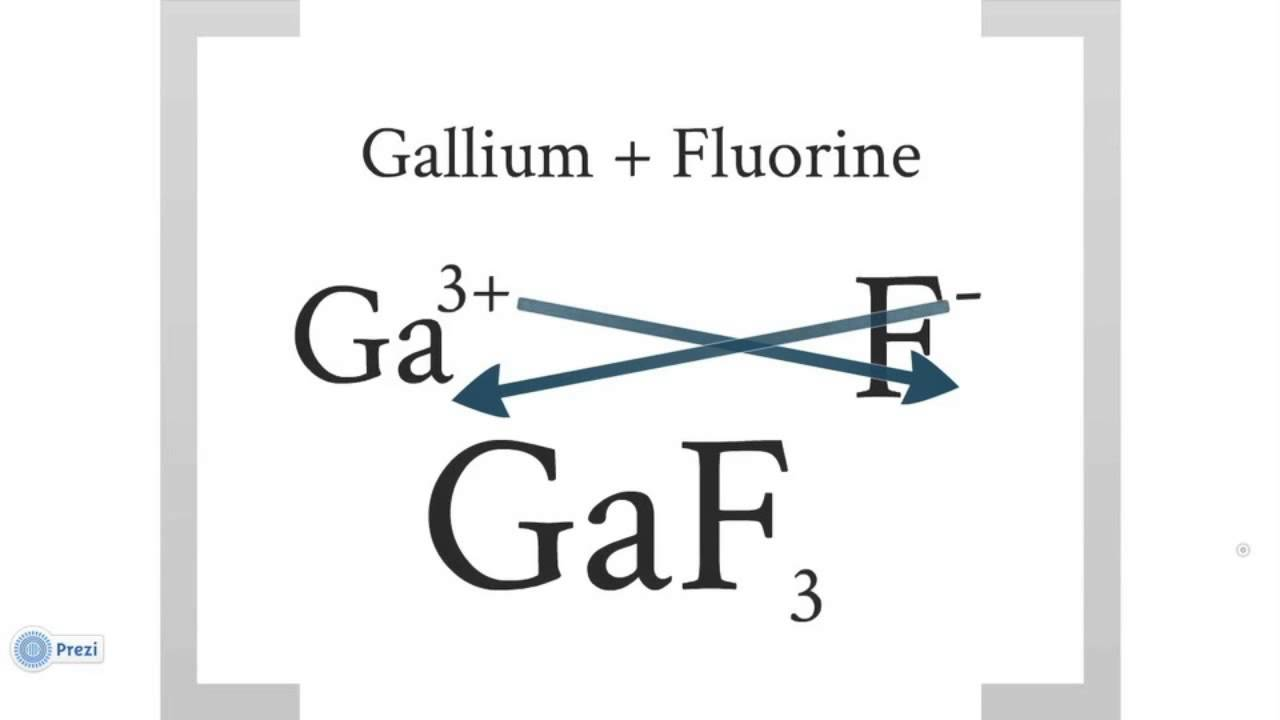Resources:

Have you found videos, websites, or explanations that helped you understand this chapter? Let us know and we'll add them to "Resources" part of this page for other students to use.

Question 1

Write the chemical formula for iron (II) carbonate.

Explanation

Iron (Fe) charge: +2

CO3 charge: -2

Cross the charges and simplifyQuestion 2

Write the chemical formula for iron (III) carbonate. For Moodle, magnesium hydrogen sulfate is written Mg(HSO4)2 meaning Mg(HSO4)2.

Explanation

Iron Charge = +3

Carbonate= -2

Cross the charges as shown in question 1

Question 3

Write the chemical formula for iron (II) chloride. (For Moodle, water is written H2O.)

Explanation

Iron Charge = +2

Chloride Charge = -1

Cross the charges as shown in question 1

Question 4

Write the chemical formula for barium hydroxide.

Explanation

Ba Charge : +2

OH Charge: -1

Cross the charges

Question 5

Write the chemical formula for ammonium sulfate.

Explanation

NH4 Charge: +1'

Sufate Charge: -2

Cross the charges.

Question 6

What is the mass of 1 mol of CH3NH2 in g?Explanation

1 mol = x g / MM CH3NH2

MM of CH3NH2 = 12 + 5(1.008) + 14 = 31

1mol= x g / 31 MM

x= 31 g

Question 7

What is the percent composition by mass of oxygen in carbon dioxide?Explanation

CO2 total mass = 44g

O2 mass: 32g

32 g /  44 g = 0.727 x 100 = 72.7%

Question 8

What is the percent composition by mass of carbon in lithium carbonate, Li2CO3?Explanation

Mass of Li2CO3 :73.891 g

Mass of Carbon: 12g

12 g / 73.891 g = .163 x 100 = 16.3%

Question 9

What is the mass of 30 molecules of nitrogen dioxide, NO2, in g? USE 3 SIG. FIG.Explanation

(14 +16(2)) MM NO2

41/(6.022*10^23) = 6.81e-23 mol = X g/ 46 MM

X= 3.1326e-21

Question 10

What is the mass of 1.38 mol NaCl in grams?Explanation

1.38 mol = X g/ MM of NaCl

MM of NaCl = 58.4428

1.38 mol = X g/ 58.4428 MM

X =80.7

Question 11

What is the mass in grams of 0.89 mol sodium phosphate, Na3PO4 ?Explanation

0 .89 mol = X g/ MM of Na3PO4

MM of Na3PO4 = 163.94

0.13 mol = X g/ 163.94 MM

X = 146

Question 12

How many moles is 188.3 g calcium carbonate, CaCO3 ?Explanation

188.3 g/ MM of CaCO3 = X moles

MM of CaCO3 = 100.0869

188.3/ 100.0869 =1.88

X = 1.88 moles

Question 13

How many moles is 105 g lithium carbonate, Li2CO3 ?Explanation

105 g / MM of Li2CO3  = X moles

MM of Li2CO3 73.891

105 / 73.891 =1.42

X = 1.42 moles

Question 14

What is the empirical formula for acetone, C2H4O2? (Keep elements in same order for your answer)

Remember to use correct symbols for elements--C for carbon (NOT c), etc.

Explanation

Question 15

A substance contains 5.00 g C and 1.70 g H. What is the empirical formula? (Answer starts with C and H is last.)

Explanation5 g C

5 g / 12 MM = .4167 mol C

1.70 g H / 1.008 MM = 1.686 mol H

1.686 mol H / .4167 = 4.05  about 4

CH4

Question 16

What is the empirical formula of a compound which is 82.64 % C by mass and 17.36 % H. (Answer starts with C and H is last.)

Explanation

Use a 100g sample...

82.64 g C / 12 MM  = 6.89

17.63 g H/ 1.008 MM = 17.49

17.49 / 6.89 = 2.5 (not a whole number) multiply it by 2 to make it a whole number

CH2.5

goes to

C2H5

Question 17

Balance the following chemical reaction using smallest whole numbers:

C3H8(g) + O2(g) --> CO2(g)+ H2O(g)

What is the number of CO2 molecules in the balanced equation?WHOOT BALANCING

Left side v Right Side

Left

• 3 mols C
• 8 mols H
• 2 mols O
Right

• 1 mol C
• 3 mol O
• 2 mol H
Lets start by balancing the H...

simply add a 4 coefficient in front of the H2O on the right

C3H8(g) + O2(g) --> CO2(g)+ 4H2O(g)

Next the Cs...

add a 3 coefficient in front of the CO2 on the right

C3H8(g) + O2(g) --> 3CO2(g)+ 4H2O(g)

Next the Os...

add a 5 coefficient in front of the O2 on the left

C3H8(g) + 5O2(g) --> 3CO2(g)+ 4H2O(g)

Now lets recheck to make sure

3 C: L and R

8 H: L and R

10 O: L and R

Question 18

What is the number of H2O molecules in the balanced equation?Question 19

What is the number of O2 molecules in the balanced equation?Question 20

Balance the following chemical reaction using smallest whole numbers:

CuO(s) +NH3(g) --> Cu(s) + N2(g) + H2O(g)

Hint, balance the elements in the following order--nitrogen, hydrogen, oxygen, and copper.

What is the number of Cu atoms in the balanced equation?after balanced its

3CuO(s) +2NH3(g) --> 3Cu(s) +N2(g) + 3H2O(g)

Question 21

What is the number of nitrogen molecules in the balanced equation? (Refer to the previous question)after balanced its

3CuO(s) +2NH3(g) --> 3Cu(s) +N2(g) + 3H2O(g)

Question 22

What is the number of water molecules in the balanced equation? (Refer to previous questions)3CuO(s) +2NH3(g) --> 3Cu(s) +N2(g) + 3H2O(g)